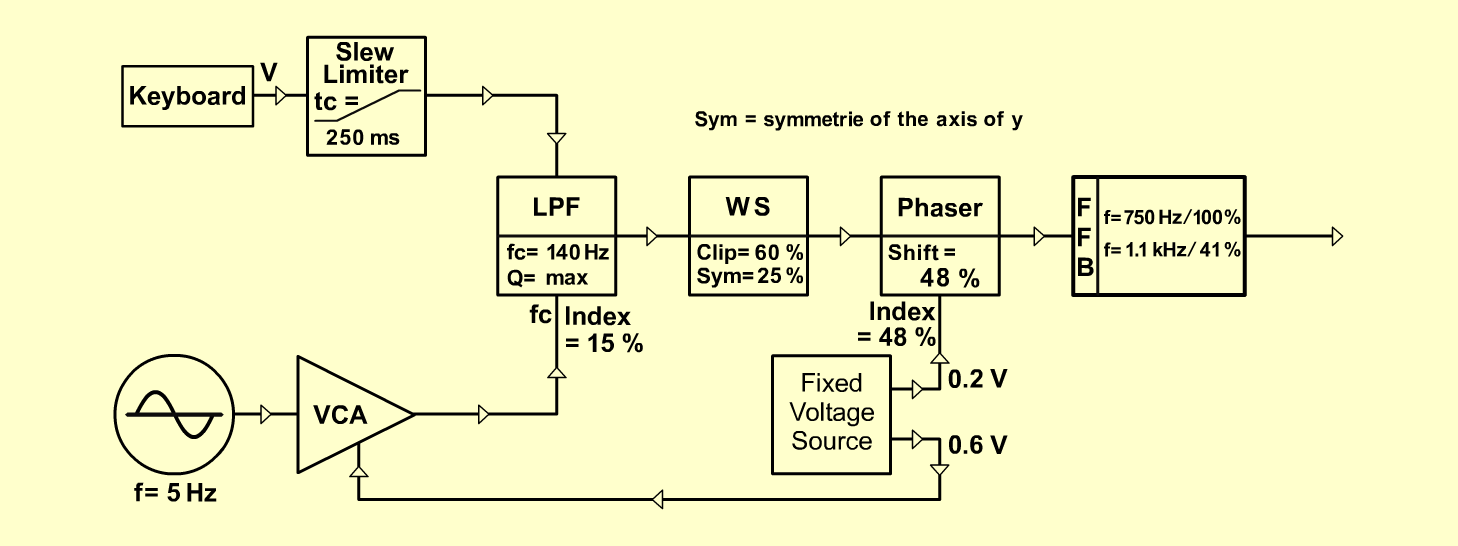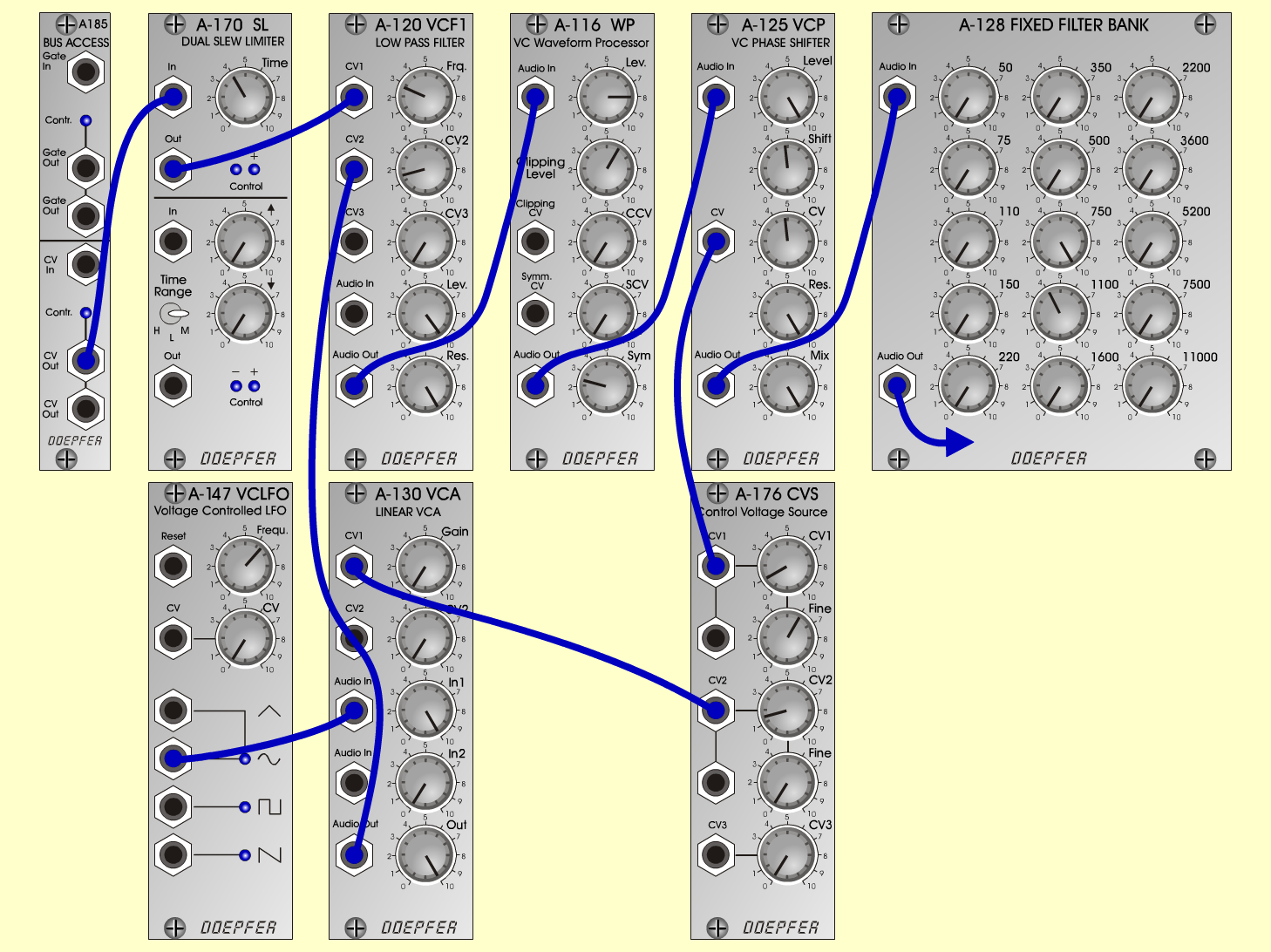Woman's VoiceVoicesD o e p f e r A - 100 Connections: Settings: A-185 (CV Out)  <=>  A-170 (In) A-170 (Out)  <=>  A-120 (CV 1) A-176 (CV 2)  <=>  A-130 (CV 1) A-147 (Sine)  <=>  A-130 (Audio In 1) A-130 (Audio Out)  <=>  A-120 (CV 2) A-120 (Audio Out)  <=>  A-116 (Audio In) A-116 (Audio Out)  <=>  A-125 (Audio In) A-176 (CV 1)  <=>  A-125 (CV) A-125 (Audio Out)  <=>  A-128 (Audio In) A-128 (Audio Out)  <=> Amplifier A-120 (Freq = 2.8, CV 2= 1.5, Lev = 9.8, Res = 10) A-116 (Lev = 8, Clip Level = 6, Sym = 2.5) A-125 (Level = 10, Shift = 4.8, CV = 4.8, Res = 10, Mix = 10) A-128 (All at 0, except:  750 = 10, 1100 = 4.1) A-170 (Time = 4) A-147 (Freq = 6.4) A-176 (CV 1 = 1, Fine = 6, CV 2 = 1.5, Fine = 0) A-130 (Gain = 0, Audio In 1 = 10,  Audio Out = 10) Notes: LP filter generates sine, which is "dirtied" by A-116 Wave Processor. Sound is then shaped by phase shifter and filter bank to get "Ah" formants. CV source controls "Ah" sound plus vibrato. VCA is used on vibrato as a gate to get finer control. Vary CV1 to get the best "Ah." Note: There is no final VCA in this loop, so sound is always on. Voice is centered around C2 on keyboard. Range is G1-A2. John A. Mitchell, April 2001Sound samples Neverglades (cutting out) Woman's Voice Woman's Voice (with reverb)